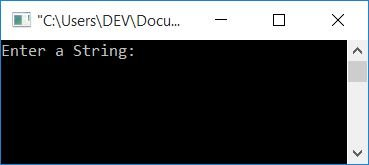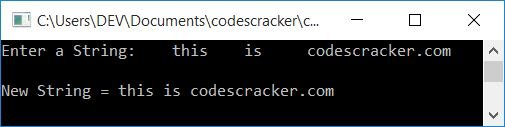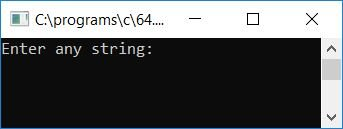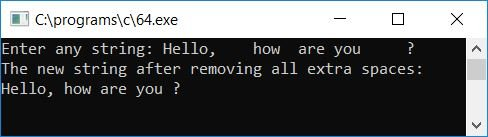# C program to remove spaces from a given string

In this article, you will learn and get code to remove spaces from strings using a C program. Here is the list of programs available in this article:

The first program removes all the spaces from a given string in C. For example, if the given string is

`Welcome To     CodesCracker`

Then the string after removing all the spaces will be:

`WelcomeToCodesCracker`

whereas the second program removes only extra spaces from the given string. For example, if the string entered by the user is:

`    this    is       codescracker`

Then, after removing only extra spaces from this string, the new string will be:

`this is codescracker`

## Remove all spaces from the string in C

To remove or delete spaces from a string in C programming, you have to ask the user to enter a string. Now start checking for spaces. If space gets found at any index, then just shift all the forward characters one index back, as shown in the program given below. The question is, "Write a program in C that removes all the spaces from a given string by the user at run-time." The answer to this question is given below:

```#include<stdio.h>
#include<conio.h>
int main()
{
char str;
int i=0, j, chk;
printf("Enter a String: ");
gets(str);
while(str[i]!='\0')
{
chk=0;
if(str[i]==' ')
{
j=i;
while(str[j-1]!='\0')
{
str[j] = str[j+1];
j++;
}
chk = 1;
}
if(chk==0)
i++;
}
printf("\nString (without spaces): %s", str);
getch();
return 0;
}```

This program was built and runs in the Code::Blocks IDE. Here is its sample run:Now supply any string and press the ENTER key to see the same string without a space. Here is the second snapshot of the sample run:#### Program Explained

Now let's discuss some of the main steps used in the above program with an example of the string shown in the previous sample run's snapshot:

• The string gets stored in the str variable in a way that
• str holds W.
• str holds e.
• str holds l.
• and so on.
• Because there are spaces, they are at index numbers 7, 10, 11, 12, 13, and 14.So
• str, str, str, str, str, str holds space.
• So when the value of i becomes 7, then the condition of the first if block evaluates to true. That is, space is discovered at the seventh index.
• So the value of i gets initialized to j, and the shifting of all forward characters (from where the space is found) to one index back gets performed. Because the space is currently found at index number 7. So
• The character at index no. 8 moved to index no. 7.
• The character at index no. 9 moved to index no. 8.
• The character at index no. 10 moved to index no. 9.
• and so on.
• until the last character of the string.
• Because the value of i is 7 right now and the character was at index no.8, it gets moved back to index no. 7.
• Therefore, here the value of i does not get incremented because, for now, the character present at index no. 7 is unknown.
• That is, we have to check it, whether it is a character or a space.
• And if no space gets found at the current index, then just increment the value of i and check for characters present at the next index number.
• Continue the process until the last character.

## Remove only extra spaces from a given string in C

Now this program only removes extra spaces from the string. That is, if there are two or more spaces available between any two words in the string, then after executing this program, there will only be one space left between all words.

```#include<stdio.h>
#include<string.h>
#include<conio.h>
int main()
{
char str;
int i, j, len;
printf("Enter a String: ");
gets(str);
len = strlen(str);
for(i=0; i<len; i++)
{
if(str==' ')
{
for(i=0; i<(len-1); i++)
str[i] = str[i+1];
str[i] = '\0';
len--;
i = -1;
continue;
}
if(str[i]==' ' && str[i+1]==' ')
{
for(j=i; j<(len-1); j++)
{
str[j] = str[j+1];
}
str[j] = '\0';
len--;
i--;
}
}
printf("\nNew String = %s", str);
getch();
return 0;
}```

Here is its sample run:Let me create the same program once more with a different approach:

```#include<stdio.h>
#include<conio.h>
#include<string.h>
int main()
{
char str, ch, count=0, len, i, j, temp;
printf("Enter any string: ");
gets(str);
len = strlen(str);
for(i=0; i<len; i++)
{
if(str[i]==32 && str[i+1]==32)
{
temp = i;
for(j=i; j<(len-1); j++)
{
str[j] = str[j+1];
}
len--;
str[len]='\0';
i = 0;
}
}
printf("The new string after removing all extra spaces:\n");
puts(str);
getch();
return 0;
}```

The above program was built and run in the Code::Blocks IDE, therefore here is the first snapshot of the sample run:Provide any string with extra spaces and press the ENTER key to see the output as shown here in the second snapshot of the sample run:Here are some of the main steps used in the above program:

• Receive any string using the gets() function inside any string variable, say str.
• Calculate the length of the string using the strlen() function and store it inside any variable, say len.
• Because indexing begins with 0, create a for loop that begins at 0 and ends at one less than the length of the string.
• Inside the for loop, check whether any character and its next character are equal to 32 or not. ASCII code 32 refers to space.
• If so, delete the next character (space) by placing each subsequent character next to the previous one.
• After removing space, reduce the string's length by one and, at the end, initialize a null-terminated character ('0') to inform the compiler that there are no more characters inside the string after that null-terminated character.
• Initialize the loop variable i with 0 and continue.
• After removing all spaces, print the value of the string as output; that will be the same string without extra spaces.

#### The same program in different languages

C Quiz

« Previous Program Next Program »